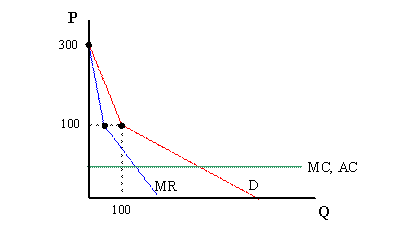### A Question per day - Day 12

1. Assume that a firm operates in Louisville and Lexington, two distinct areas of the State.

If this firm allowed its branches to operate independently, then the demand and marginal revenue for its product in Louisville (q1 = output in Louisville) and Lexington (q2 = output in Lexington) respectively would be:

 Louisville Lexington P = 100 ñ 0.5q1 P = 300 ñ 2q2 MR = 100 ñ q1 MR = 300 ñ 4q2

In both markets, the marginal and average cost are constant, and assumed to be:

MC = AC = 20

When this firm makes a central decision on behalf of both firms (i.e. doesnít treat these markets separately, but charges the same price in both markets), it uses the following demand and marginal revenue curves (Q = market output = q1 + q2):

 Overall Demand: Overall Marginal Revenue: P = 300 ñ 2Q if Q < 100 MR = 300 ñ 4Q if Q < 100 P = 140 ñ 0.4Q if Q ≥ 100 MR = 140 ñ 0.8Q if Q ≥ 100

The overall market graph would look something like this:Assume that this monopoly firm currently charges the same price in both markets, a price obtained from its use of the linear pricing approach discussed in class.

Show whether this firm can use third degree price discimination to increase profits.

2. Assume that a monopoly firm faces the following demand and cost functions:

(Demand) P = 1000 - 2Qd
(Marginal Revenue) MR = 1000 - 4Qd
(Marginal Cost) MC = 200
(Average Cost) AC = 200

What is the (most) potential profit available from using a two-part tariff pricing strategy?